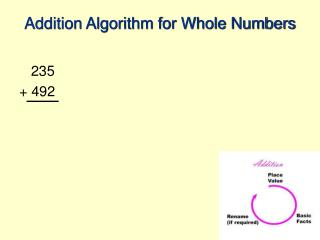DownloadDownload Presentation# Addition Algorithm for Whole Numbers

Download Presentation## Addition Algorithm for Whole Numbers

- - - - - - - - - - - - - - - - - - - - - - - - - - - E N D - - - - - - - - - - - - - - - - - - - - - - - - - - -
##### Presentation Transcript

1. Addition Algorithm for Whole Numbers 235 + 492

2. Addition Algorithm for Whole Numbers 235 + 492 7 Place Value : Start with smallest pieces (ones) Basic Fact: 5 ones + 2 ones = 7 ones Basic Fact: 5 + 2 = 7 Rename: not needed

3. Addition Algorithm for Whole Numbers 1 235 + 492 27 Place Value: move to the tens Basic Fact: 3 tens + 9 tens = 12 tens Basic Fact: 3 + 9 = 12 Rename: 12 tens as 1 hundred + 2 tens

4. Addition Algorithm for Whole Numbers 1 235 + 492 727 Place Value: move to the hundreds Basic Fact: 1 hundred + 2 hundreds + 4 hundreds = 7 hundreds Basic Fact: 1 + 2 + 4 = 7 Rename: not needed

5. Addition Algorithm for Whole Numbers 235 + 492 200 + 30 + 5 400 + 90 + 2

6. Addition Algorithm for Whole Numbers 235 + 492 7 200 + 30 + 5 400 + 90 + 2 7

7. Addition Algorithm for Whole Numbers 1 235 + 492 27 200 + 30 + 5 400 + 90 + 2 120 + 7

8. Addition Algorithm for Whole Numbers 100 200 + 30 + 5 400 + 90 + 2 20 + 7 1 235 + 492 27

9. Addition Algorithm for Whole Numbers 100 200 + 30 + 5 400 + 90 + 2 700 + 20 + 7 1 235 + 492 727

10. Notice the cycle For all addition algorithms there is a cycle of: • Place Value (identify like sized pieces) • Basic Facts • Rename (if necessary)